# How to round a formula result in Excel

You can watch a video tutorial here.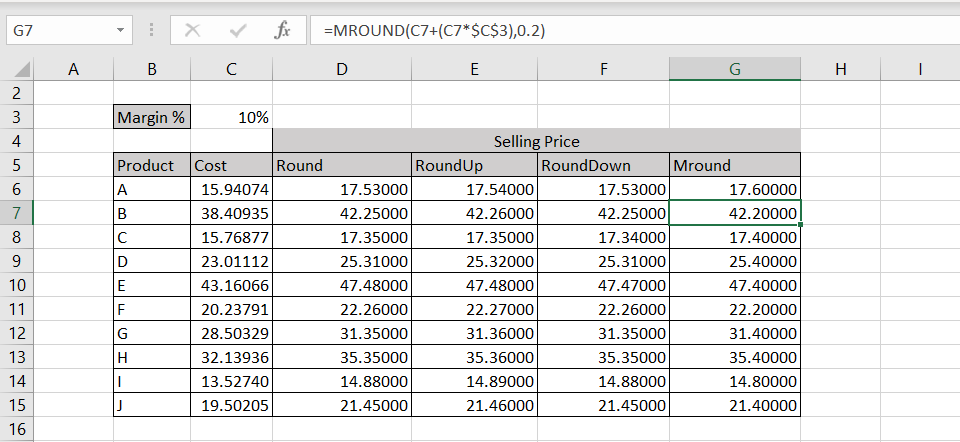Excel is frequently used for calculations and has many functions to help with basic mathematical operations.  When using formulas to create a calculated column, you may need to round the result if there are too many decimal places. Excel has round functions that can be used in combination with the formula to round the result and reduce the number of steps for calculation.

### Option 1 – Use the ROUND() function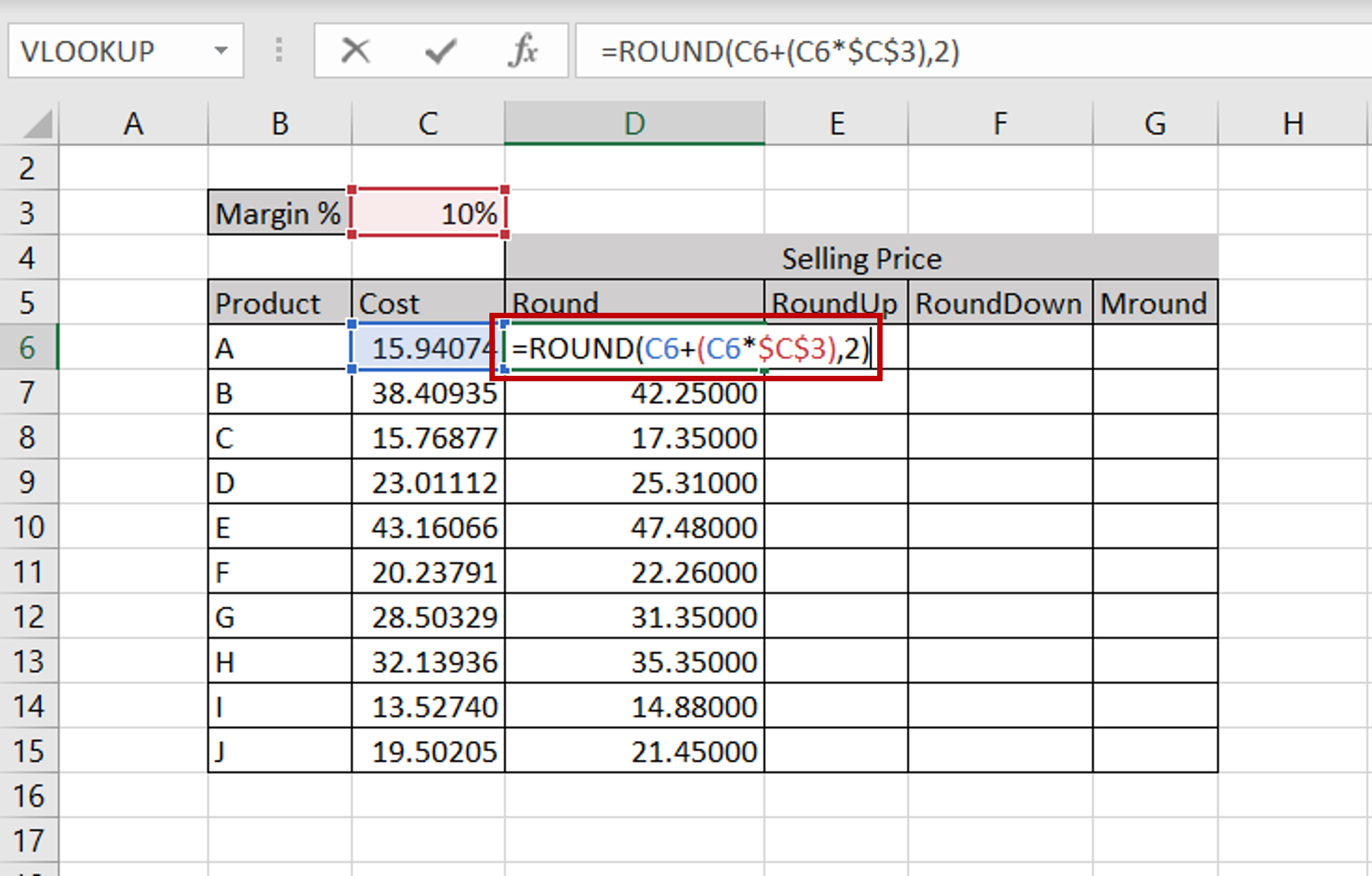– Select the cell that has the formula
– Edit the formula by adding the ROUND function and giving the number of decimal places:
=round(<formula>,2)
– Copy the formula to the rest of the cells
– The numbers are rounded (either up or down, depending on the value of the number),  to 2 decimal places

### Option 2 – Use the ROUNDUP() function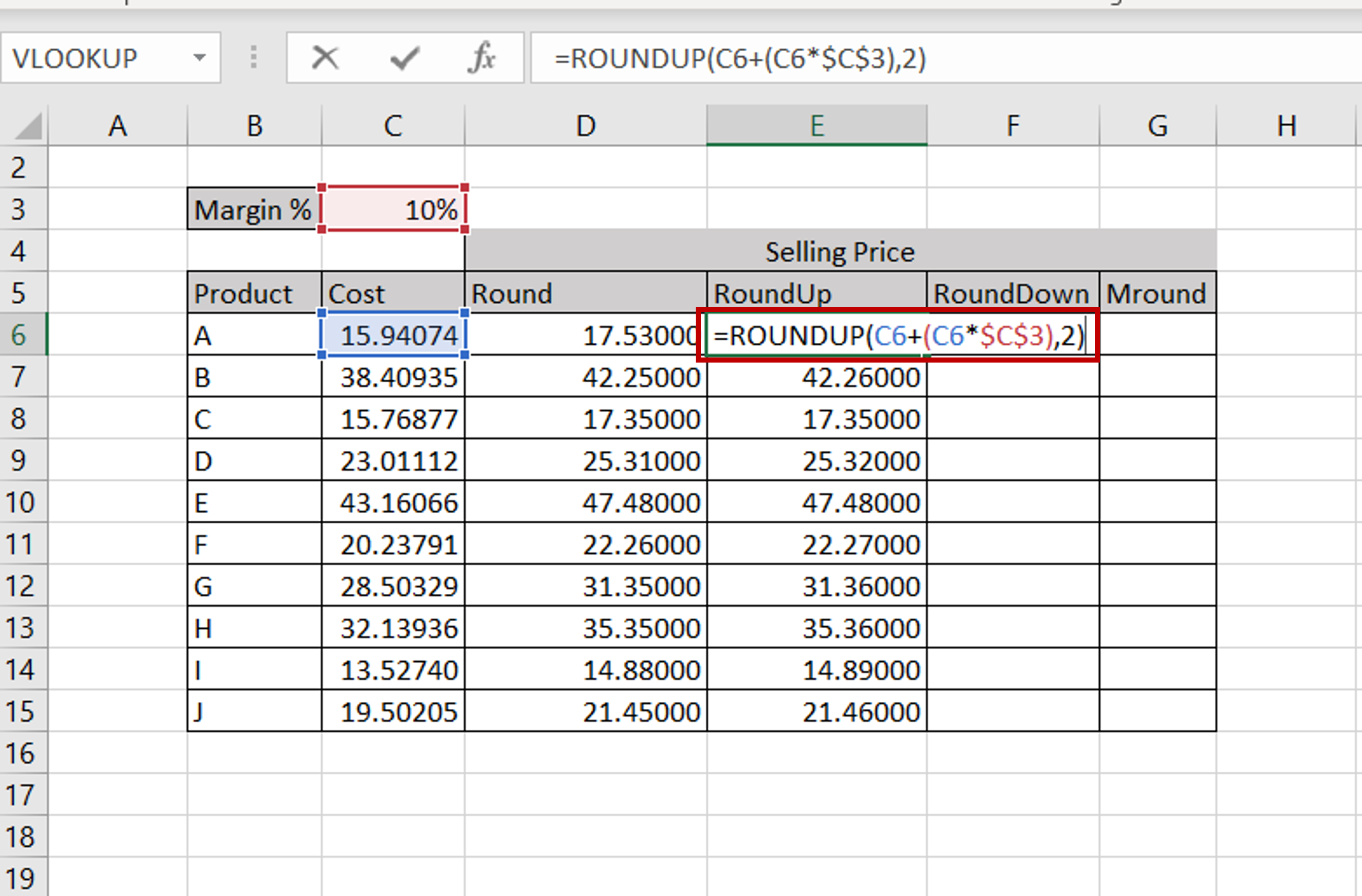– Select the cell that has the formula
– Edit the formula by adding the ROUNDUP function and giving the number of decimal places:
=roundup(<formula>,2)
– Copy the formula to the rest of the cells
– The numbers are rounded up to 2 decimal places

### Option 3 – Use the ROUNDDOWN() function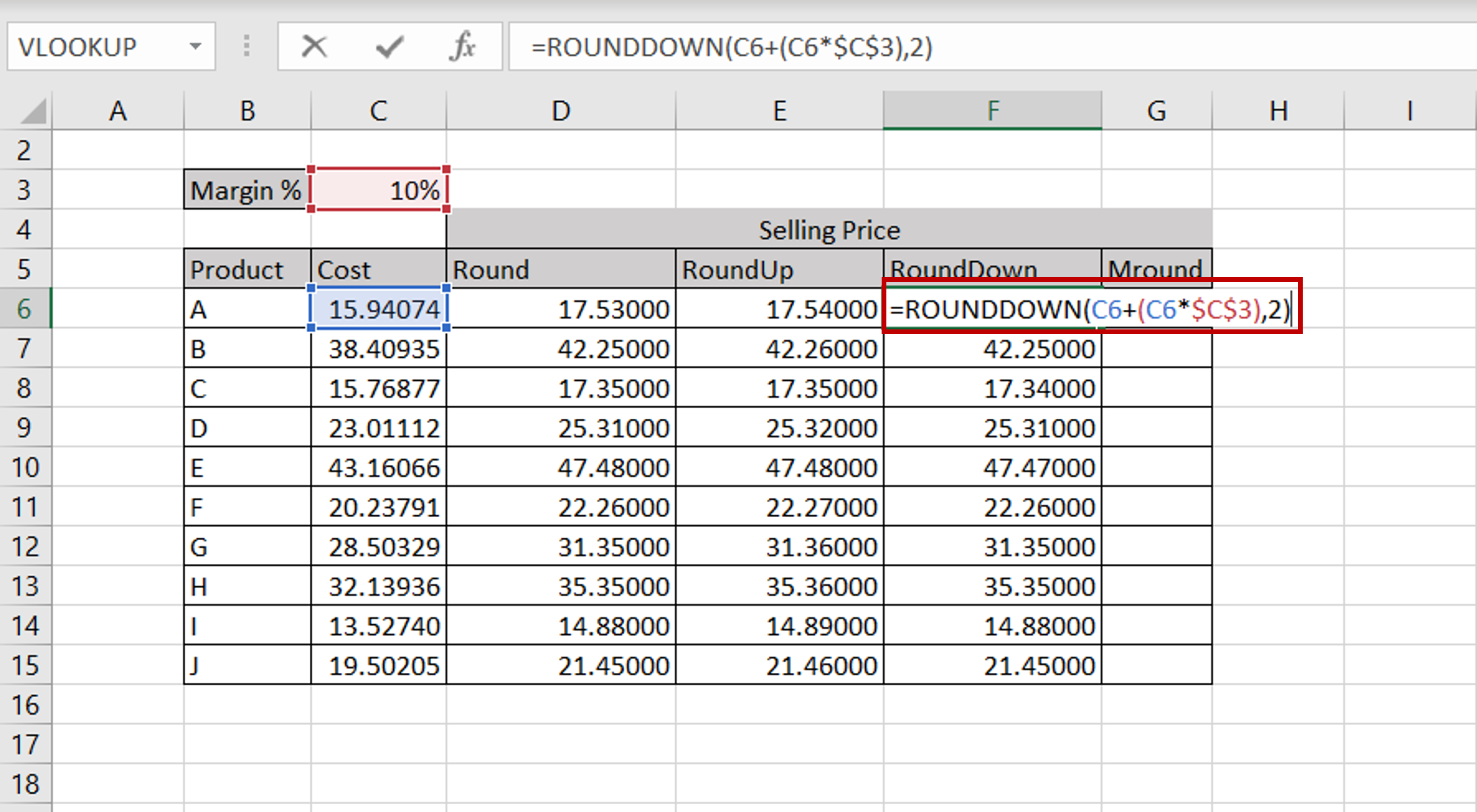– Select the cell that has the formula
– Edit the formula by adding the ROUNDDOWN function and giving the number of decimal places:
=rounddown(<formula>,2)
– Copy the formula to the rest of the cells
– The numbers are rounded down to 2 decimal places

### Option 4 – Use the MROUND() function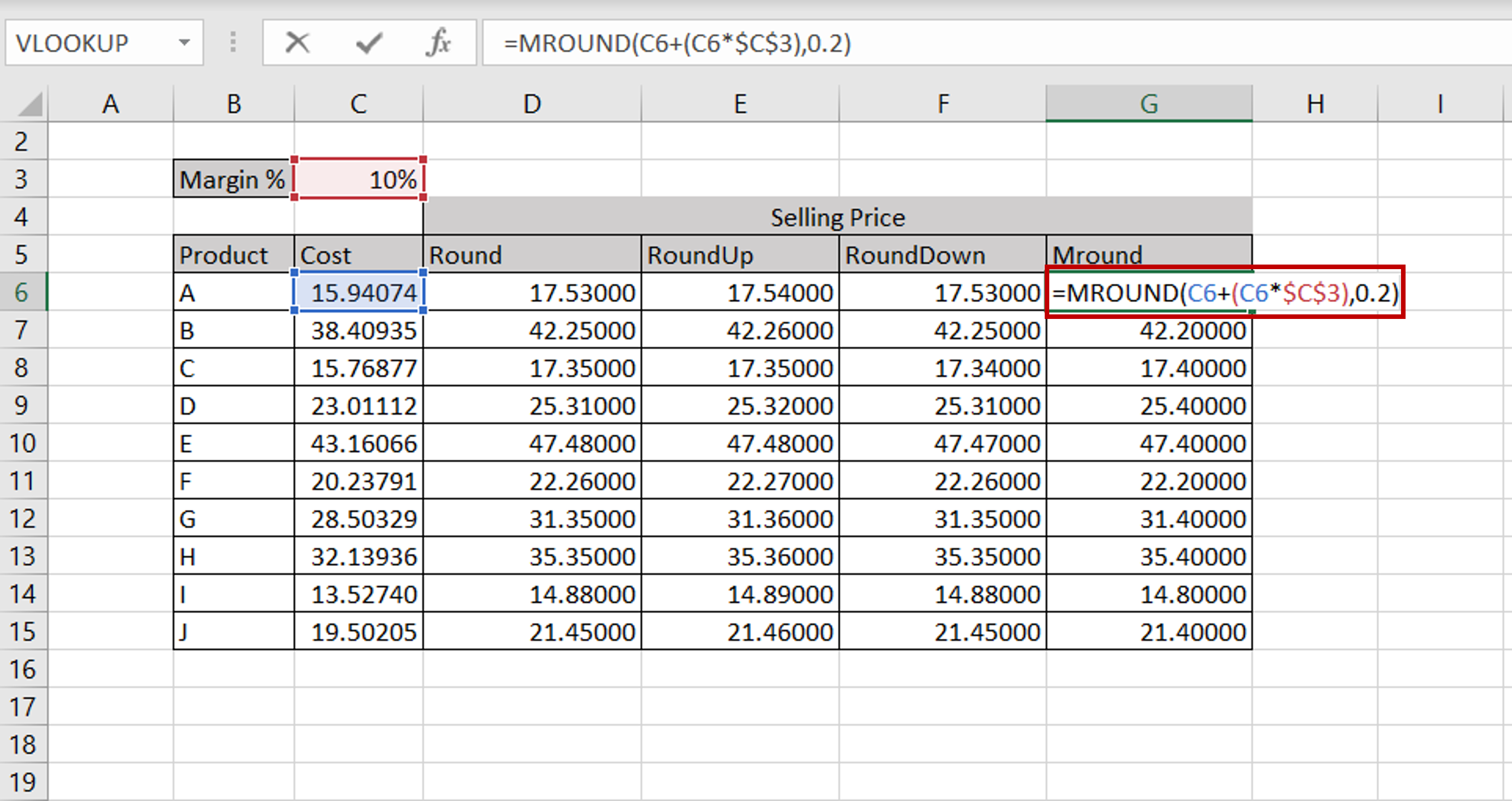– Select the cell that has the formula
– Edit the formula by adding the MROUND function and giving the multiple to which the number is to be rounded:
=roundup(<formula>,0.2)
– Copy the formula to the rest of the cells
– The numbers are rounded to 0.2 precision